# Naming Three Dimensional Shapes

You might already be familiar with some of the three dimensional shapes.

Check out these examples: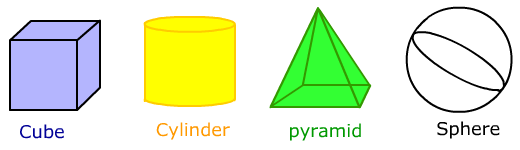To name some of the three dimensional shapes you are not familiar with, we will start by separating the shapes into two basic groups.

Prisms

2 congruent and parallel bases

Lateral sides are parallelograms
Pyramids

Only 1 base

Lateral sides are triangles

The lateral sides meet together at an apex

The names of the prisms and pyramids are based on the bases. Here are some examples.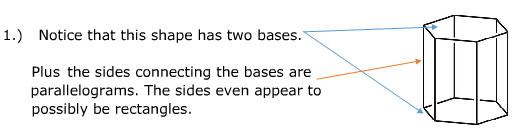From these two characteristics we can tell that the shape is a prism. Now we need to know what kind of prism.

Check out the base. It has 6 sides. So the base is a hexagon.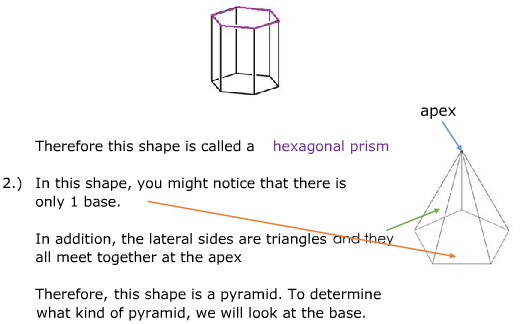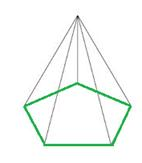The base has 5 sides. Therefore, the base is a pentagon. That makes this shape a pentagonal pyramid.

3.)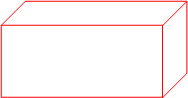All of the sides are rectangles in this shape. Any of the sides could be considered the base. The sides are rectangles and there are two bases no matter which side you pick. Therefore, this is a rectangular prism.

4.)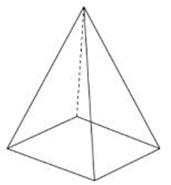This shape only has one base in the shape of a rectangle. The lateral sides are triangles. Therefore, this shape is a rectangular pyramid.

Let's Review

There are two groups of three dimensional shapes that you can easily name. These include the prisms and the pyramids. Prisms have two parallel bases and the lateral sides that connect the bases are parallelograms.

Pyramids have one base and the lateral sides are triangles. The lateral sides meet together at an apex.

To name the object, determine if the shape is a prism or pyramid. Then determine the name of the base. Put these together to form the name. Some examples include triangular pyramid, octagonal prism, dodecagonal prism, etc.

 Related Links: Math Geometry Match Geometric Shapes - Game Polygon Shapes Quadrilaterals Shapes